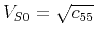On anelliptic approximations for qP velocities in TI and orthorhombic mediaNext: Discussion Up: Sripanich & Fomel: qP Previous: Examples

# Application to wave extrapolation

One possible application of the proposed phase-velocity approximations (equations 23 and 45) is seismic wave extrapolation based on the anisotropic wave equation. Fomel et al. (2013) and Sun and Fomel (2013) presented a lowrank approximation method to accomplish this task. The proposed phase-velocity approximations (both in TI and orthorhombic media) are converted to their corresponding dispersion relations involving frequency and wavenumber and incorporated into the wave extrapolator formulated in the Fourier domain. An example of wave extrapolation in the complex BP 2007 TTI model is shown in Figure 11. The same portion of the model was investigated by Fomel et al. (2013) and Sun and Fomel (2013). For simplicity, we take the shear-wave velocity () to be. The results are shown in Figure 11 and demonstrate noticeably smaller phase errors obtained from the proposed approximation.snaptssum-0,snaptssum-1,errortssum-2,errortssum-1
Figure 11.
Multiple snapshots and errors of the wavefield extrapolation results for the BP TTI 2007 model. a) Wavefield extrapolation using exact phase velocity. b) Wavefield extrapolation using proposed phase-velocity approximation (23). c) Absolute error in-plane of the acoustic approximation. d) Absolute error in-plane of the proposed approximation (six-parameter).An example in a heterogeneous tilted orthorhombic medium is shown in Figures 12 and 13 using the parameters of the tilted orthorhombic model from Song and Alkhalifah (2013). In this model, the anisotropic parameters in the notation of Alkhalifah (2003) are specified in the range,m/s,m/s,m/s,,, and. The exact formulas for,, andare(62)(63)(64)

where,, andare components in the model. These values of parameters correspond to,,,,, and. The model, according to the right-hand rule is rotatedcounterclockwise around theaxis and subsequentlycounterclockwise around theaxis. We perform wave extrapolation using the exact dispersion relation and compare it with the results from the proposed approximation (equation 45), as well as the weak-anisotropy approximation (Tsvankin, 1997), and the acoustic approximation (Song and Alkhalifah, 2013; Alkhalifah, 2003). The error plots shown in Figure 13 demonstrate noticeably smaller phase errors from the proposed approximation.wave0,wave1
Figure 12.
Wavefield extrapolation results in an example of the tilted orthorhombic model. a) Wavefield extrapolation using the exact phase velocity. b) Wavefield extrapolation using the proposed phase-velocity approximation (equation 45). The wavefields are virtually identical.error3,error2,error1,error4
Figure 13.
Errors in wavefield extrapolation results in an example of the tilted orthorhombic. a) Absolute error of the weak-anisotropy phase-velocity approximation. b) Absolute error of the acoustic phase-velocity approximation. c) Absolute error of the proposed phase-velocity approximation (six-parameter). d) Absolute error of the proposed phase-velocity approximation (nine-parameter).On anelliptic approximations for qP velocities in TI and orthorhombic mediaNext: Discussion Up: Sripanich & Fomel: qP Previous: Examples

2017-04-14